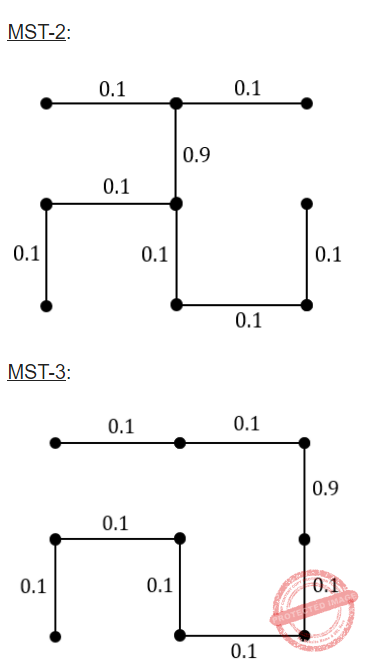October 14, 2023
October 14, 2023
October 14, 2023
###### Teaching Aptitude
October 14, 2023
 Question 19
Consider the following undirected graph with edge weights as shown:The number of minimum-weight spanning trees of the graph is _______
 A 3
Question 19 Explanation:

To find the number of spanning trees using 2 methods:

1. If graph is complete, use n^n-2 formula
2. If graph is not complete, then use kirchhoff theorem.

Steps in Kirchoff’s Approach:

(ii) Replace all non-diagonal is by – 1.

(iii) Replace all diagonal zero’s by the degree of the corresponding vertex.

(iv) Co-factors of any element will give the number of spanning trees.

Using the Kirchhoff theorem will take lot of time because the number of vertices are 9.

So, we use brute force technique to solve the problem with the help of Kruskal’s algorithm.Question 19 Explanation:

To find the number of spanning trees using 2 methods:

1. If graph is complete, use n^n-2 formula
2. If graph is not complete, then use kirchhoff theorem.

Steps in Kirchoff’s Approach:

(ii) Replace all non-diagonal is by – 1.

(iii) Replace all diagonal zero’s by the degree of the corresponding vertex.

(iv) Co-factors of any element will give the number of spanning trees.

Using the Kirchhoff theorem will take lot of time because the number of vertices are 9.

So, we use brute force technique to solve the problem with the help of Kruskal’s algorithm.